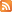Entries (RSS) -Comments (RSS)

### Chapter 3 Stash It 2011

Hello again my lovelies. Here is your chapter 3 stash it assignment. You will need to use your 11 x 17 yellow papers to show all of the exponent laws. Make sure you have all of the following laws: product law, quotient law, power of a power law, power of a product, power of a quotient law, power of zero law, power of one law, base of one law.
For each law show an algebraic expression describing the law, write the "rule" for the law and show an example of the law. Include a word problem if applicable. (10 marks)

Your self assessment will show that you can do all the work that is not included in the "rules" sheet. They will include things like: I can evaluate powers that include or do not include parantheses; I can identify the error in a simplification of an expression (from the textbook); I can use the order of operations on expressions with powers; I can apply the laws of exponents; I can determine the sum of powers; i can determine the difference of two powers; I can identify the error in applying the order of operations in an incorrect solution; I can solve problems taht require combining powers; and I can use powers to solve problems that involve repeated multiplication. (5 marks)

Also include in the stash it your journal that is up to date. In your journal highlite a best journal entry. Tell me why you think it is a best journal entry. (4 marks)

Include also tests: Unit Two Final Test, Unit Three Final Test make sure they are signed by a parent.(1 marks)

This Stash It is due on December 12.

### Chapter 2 Stash It Assignment

Chapter 2 Stash It Assignment 2011

In this chapter we worked with rational numbers. For your assignment you will have completed the following:

Blogged 2 questions.
Commented on at least 8 other questions. (4 marks)

Complete a Venn diagram showing our Real number system with definitions of each kind of number, its abbreviation, and examples. (4 marks)

Self assessment Stop Light Activity with examples to show that you know.
For Stop Light 2.2: “I can perform operations on rational numbers in decimal form.” You must show one of each of the following operations: +, -, ×, ÷
Each of the operations must demonstrate knowledge of using positives and negatives. (4 marks)

For Stop Light 2.2: “I can perform operations on rational numbers in fraction form.” You must show one of each of the following operations: +, -, ×, ÷
Each of the operations must demonstrate knowledge of using positives and negatives, and how to convert between mixed and improper fractions. (4 marks)

Problems of the Week question 3 and 4. (4 marks)

### Grade 9 October 20-24, 2011

Hello all students in grade 9 for 2011 to 2012. Here is your homework for this weekend. Do question 4 and 5 in your math text book on page 60. Complete Mangahigh Challenges and Studyladder work as well.

For all students who have yet to hand in their Stash-It ... get it done.

Some students still owe me a post... you know who you are... get it done.

Have a great weekend.

### Math Words For Chapter 10

Please put the following words into a foldable give a definition, draw a picture and a not picture. These are the words: minor arc, major arc, perpendicular bisector, tangent, chord, inscribed angle, central angle and subtend.

### Work to be handed in for Unit 5

Dear students,

For unit five I would like you to submit an electronic Stash-it. In it you will have all the words from the unit described and illustrated. Give "not" examples if it applies. For instance showing that x and x squared are not like terms.

As well I would like you to hand in your work for the Kayak for Rent Challenge problem from page 204 in your textbook,electronically.

The last thing I want you to do is give examples for the Outcomes for Chapter 5 to show you can do the work, from your self assessment. Also tell the colour you are for that outcome.Example:

5.1 I can use mathematical terminology to describe polynomials:green x monomial, -2x + 5, binomial, 3x + 7y - 4, trinomial, 2a - 3b - 5c -7 polynomial, etc.

I would like this work submitted to me at rbacke@wsd1.org no later than Friday January 21, 2011. As usual if you have any questions see me.